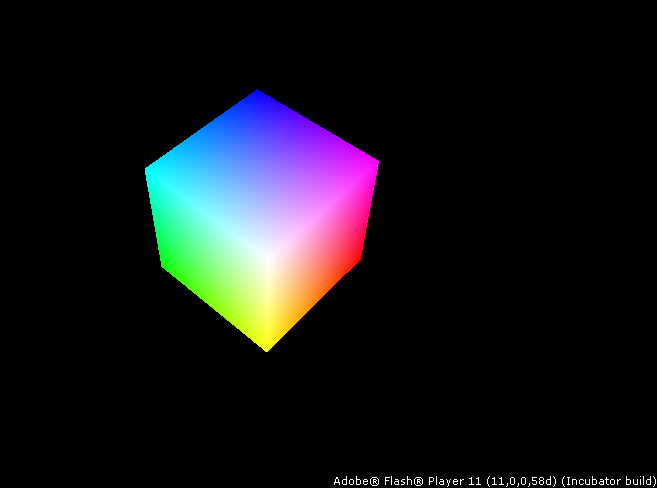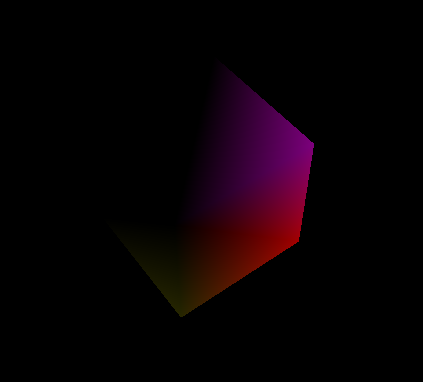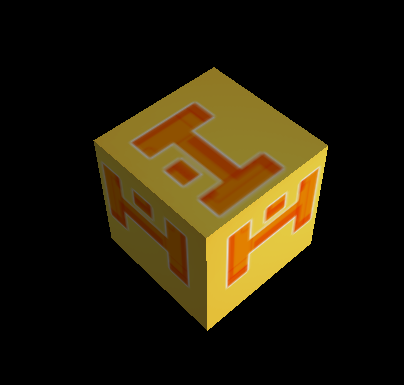# Using Flash 3D API

Here's a small tutorial explaining how to use the latest Flash Player 11 3D APIs with Haxe.

# Installation

• Flash Player 11+ from Adobe
• Install Haxe 2.10 Release
• Once Haxe is installed, run the following commandline to install the haxe `format` library :
`haxelib git hxsl git://github.com/ncannasse/hxsl.git`

# Example 0 - Cube

You can test this example by running `haxe test.hxml` or simply opening the prebuild `test.swf` inside your browser. Make sure you have Flash Player 11 Installed !Let's look at `Test.hx` in details :

In the `new` method (Haxe class constructor), we will setup a new 3D stage that will be the place our 3D rendering occur. This is done by the following lines :

```var s : flash.display.Stage3D;
//...
stage = flash.Lib.current.stage;
s = stage.stage3Ds;
s.requestContext3D();```

When the 3D context is ready, we will configure it and allocate a new Shader, a new Camera, and the 3D Cube polygon data :

```var c : flash.display3D.Context3D;
var pol : Polygon;
var camera : Camera;
// ...
c = s.context3D;
c.enableErrorChecking = true;
c.configureBackBuffer( stage.stageWidth, stage.stageHeight, 0, true );

camera = new Camera();

pol = new Cube();
pol.alloc(c);
}```

Then in the `update` method, we will start by doing some setup for the context 3D :

```c.clear(0, 0, 0, 1);
c.setDepthTest( true, flash.display3D.Context3DCompareMode.LESS_EQUAL );
c.setCulling(flash.display3D.Context3DTriangleFace.BACK);```

Then handle the camera movement, which can be controlled with arrow keys and keypad +/- keys :

```if( keys[K.UP] )
camera.moveAxis(0,-0.1);
if( keys[K.DOWN] )
camera.moveAxis(0,0.1);
if( keys[K.LEFT] )
camera.moveAxis(-0.1,0);
if( keys[K.RIGHT] )
camera.moveAxis(0.1, 0);
if( keys )
camera.zoom /= 1.05;
if( keys )
camera.zoom *= 1.05;
camera.update();```

Once this is done, we can access the camera projection matrix and create a model-position matrix for our cube which will rotate around its Z-axis :

```var project = camera.m.toMatrix();
var mpos = new flash.geom.Matrix3D();
mpos.appendRotation(t * 10, flash.geom.Vector3D.Z_AXIS);```

What's left is simply to setup our shader, draw our polygon, then display the 3D context :

```shader.mpos = mpos;
c.drawTriangles(pol.ibuf);
c.present();```

This will show us a rotating 3D Cube. Please note that colors are representing the cube vertex position, so going from (0,0,0) = black to (1,1,1) = white.

One part that we didn't cover was how the cube get transformed and colored. This is done by a shader. Unlike AS3 where you would write your shader in assembler, Haxe shaders are using HxSL - Haxe Shader Language and are directly written in Haxe, as you can see at the beginning of your `Test.hx` program :

```class Shader extends hxsl.Shader {
static var SRC = {
var input : {
pos : Float3,
};
var color : Float3;
function vertex( mpos : M44, mproj : M44 ) {
out = pos.xyzw * mpos * mproj;
color = pos;
}
function fragment() {
out = color.xyzw;
}
}
}```

The `SRC` section contains Haxe code which is compiled at the same time you're compiling your project by using Haxe Macros.

# Example 1 - Lighting

In this example we will light our 3D Cube using a simple Gouraud algorithm. In order to do that we will add normals to our cube geometry, by adding the following line after creating the polygon :

`pol.addNormals();`

This will calculate a per-vertex normal. Since the cube points are shared by triangles faces, you'll get very smooth normals.

Now that we have normals, let's create a rotating light and pass it to our shader parameters :

```var light = new flash.geom.Vector3D(
Math.cos(t * 10) * 1,
Math.sin(t * 5) * 2,
Math.sin(t) * Math.cos(t) * 2
);
light.normalize();
// ...

Once this is done, we simply have to modify our shader code. In order to calculate the light power, we make a dot product between the transformed normal and the light direction. We make sure that power is not negative and we apply this 0-1 factor to the per-vertex color :

```var tnorm = (norm * mpos).normalize();
var lpow = light.dot(tnorm).max(0);
color = pos * lpow;```If you want to have more orthogonal normals, you can simply ensure that each vertex will be have an unique normal by adding just before normals calculation the following line :

`pol.unindex();`

# Example 2 - Texturing

In this example, we will add a texture. For this we need first to add texture coordinates to our Cube data :

`pol.addTCoords();`

Please note that texture coordinates will require un-indexed cube points.

Then we will create an embedded texture from the local files and allocate in our 3D context :

```@:bitmap("hxlogo.png")
class HaxeLogo extends flash.display.BitmapData {
}
// ...
var logo = new HaxeLogo();
texture = c.createTexture(logo.width, logo.height, flash.display3D.Context3DTextureFormat.BGRA, false);

We will pass the texture to our shader parameters as well :

`shader.tex = texture;`

Now what's left is to simply modify our shader so it will read the color directly from the texture by using the texture coordinates we have stored in our geometry :

```var input : {
pos : Float3,
norm : Float3,
uv : Float2,
};
var tuv : Float2;
var lpow : Float;
function vertex( mpos : M44, mproj : M44, light : Float3 ) {
out = pos.xyzw * mpos * mproj;
var tnorm = (norm * mpos).normalize();
lpow = light.dot(tnorm).max(0);
tuv = uv;
}
function fragment( tex : Texture ) {
out = tex.get(tuv) * (lpow * 0.8 + 0.2);
}```Please note that we are applying some operations on our 0-1 light power factor. In that case we are adding a 20% ambient light to make sure that the texture colors will not turn completely black when they don't face the light.

version #15782, modified 2012-12-10 00:32:43 by ncannasse